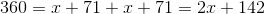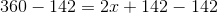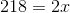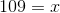# ISEE Upper Level Math : How to find an angle in a parallelogram

## Example Questions

### Example Question #9 : Parallelograms

Solve for: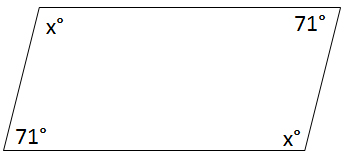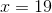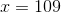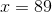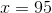Explanation:

Find the sum of the interior angles of the polygon using the following equation where n is equal to the number of sides.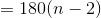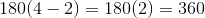The sum of the angles must equal 360.